# CAT 2019 Question Paper | Quants Slot 2

###### CAT Previous Year Paper | CAT Quants Questions | Question 1

The best questions to practice for CAT Exam are the actual CAT Question Papers. 2IIM offers you exactly that, in a student friendly format to take value from this. In CAT 2019 we saw some beautiful questions that laid emphasis on Learning ideas from basics and being able to comprehend more than remembering gazillion formulae and shortcuts. Original CAT Question paper is the best place to start off your CAT prep practice. This page provides exactly that. To check out about 1000 CAT Level questions with detailed video solutions for free, go here: CAT Question Bank

Question 1 : The real root of the equation 26x + 23x+2 - 21 = 0 is

1. $$frac{log_{2}3}{3}$ 2. log29 3. $\frac{log_{2}7}{3}$ 4. log227 ## 🚀 Introductory Offer! Buy CAT'21 95-99 Booster Course for just Rs. 4500 Valid until 19th Sep #### 2IIM : Best Online CAT Coaching. ### Video Explanation ## Best CAT Coaching in Chennai #### CAT Coaching in Chennai - CAT 2021Online Batches Available Now! ### Explanatory Answer Given equation - 26x + 23x+2 - 21 = 0 The idea is to convert the above equation to a quadratic one. So, 26x =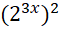Let y =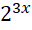Now, 26x + 23x+2 - 21 = 0 can be rewritten as+ 22.23x - 21 = 0 y2 + 4y - 21 = 0 Solving the above quadratic equation,$y + 7) (y - 3) = 0
So, y = -7 or +3
y ==> y should always be positive
Therefore, y = -7 is not a valid solution. So, only y = +3 exists.= 3
Taking log on both sides,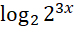=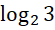3x =x =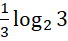, which is the real solution to the given equation

The question is "The real root of the equation 26x + 23x+2 - 21 = 0 is"

##### Hence, the answer is $$frac{log_{2}3}{3}$ Choice A is the correct answer. ###### 🚀 Introductory Offer! Buy CAT'21 95-99 Booster Course for just Rs. 4500. Valid until 19th Sep ###### Already have an Account? ###### CAT Coaching in ChennaiCAT 2021Enroll at 49,000/- 44,000/- Online Classroom Batches Starting Now! ###### Best CAT Coaching in Chennai Introductory offer of 5000/- Attend a Demo Class ###### Best Indore IPM & Rohtak IPM CoachingSignup and sample 9 full classes for free. Register now! ##### Where is 2IIM located? 2IIM Online CAT Coaching A Fermat Education Initiative, 58/16, Indira Gandhi Street, Kaveri Rangan Nagar, Saligramam, Chennai 600 093 ##### How to reach 2IIM? Phone:$91) 44 4505 8484
Mobile: (91) 99626 48484 / 94459 38484
WhatsApp: WhatsApp Now
Email: info@2iim.com